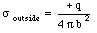## Solutions Homework Set # 2 - Physics 122

Problem 1.

Consider a cylindrical Gaussian surface of radius R (where R is larger than the radius r of the insulator) and length L. Because of the symmetry of the charge distribution, the electric field will be directed along the radial direction (perpendicular to the symmetry axis of the insulator). Assume that the magnitude of the electric field at radius R is E(R). The electric flux through this Gaussian surface is equal to: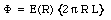The charge that is enclosed by the Gaussian surface is equal to:where [rho] is the charge density of the insulator. Applying Gauss' Law we conclude that: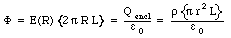The electric field E(R) is thus equal to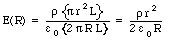Problem 2.

The calculation of the electric field inside the insulator is very similar to the procedure used in problem 1. Consider again a Gaussian surface of radius R (where now R is smaller than the radius r of the insulator). The electric flux though this Gaussian surface is equal toThe charge that is enclosed by the Gaussian surface is equal to: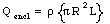Applying Gauss' Law we conclude that: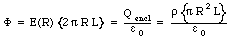The electric field E(R) is thus equal to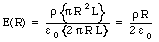Problem 3.

In this problem we are again considering the electric field outside the charge distribution on the insulator. In this case the charge distribution is that of a line charge with the same effective charge per unit length as that of the insulator considered in Problems 1 and 2. The effective line charge of the insulator in Problems 1 and 2 (the charge per unit length) is equal to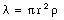The procedure used to calculate the electric field at a distance R from the wire is very similar to the procedure used in Problem 1. Consider a cylindrical Gaussian surface of radius R (where R is larger than the radius r of the insulator) and length L. The electric flux through this Gaussian surface is equal to:The charge that is enclosed by the Gaussian surface is equal to: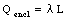Applying Gauss' Law we conclude that: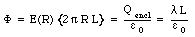The electric field E(R) is thus equal to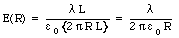Problem 4.

The dipole moment d of the charge distribution with respect to charge -q is equal to: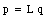where L is the distance between the opposite charges. The dipole moment is directed from the negative charge to the positive charge

Problem 5.

The torque experienced by the dipole as a result of the electric field E is equal to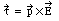The magnitude of the torque is equal to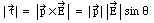Problem 6.

The net force acting on the dipole is equal to the sum of the forces acting on the individual charges. Since the electric field is uniform, the force exerted on the positive charge is equal in magnitude to the force exerted on the negative charge, but pointed in the opposite direction. The net force acting in the system is thus equal to 0 N.

Problem 7

The symmetry of the problem immediately implies that electric field is directed radially away from the axis of the cylinder. The magnitude of the electric field is only a function of the distance r from the axis.

Consider a cylindrical Gaussian surface with radius r and length L. The electric flux through this surface is equal to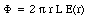Applying Gauss' law we obtain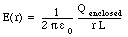For r < a, the enclosed charge is equal to 0. Thus, the electric field is also equal to 0.

Problem 8

Since the electric field is parallel to the x axis, the only surfaces of the cube that contribute to the total flux are those that are perpendicular to the x axis. There are only two of such surfaces. The surface located at x = 0 in the y-z plane is perpendicular to the x axis, but the electric field at its surface is equal to 0, and the total flux through this surface is thus also equal to 0. The second surface that is perpendicular to the x-axis is the surface located at x = a, parallel to the y-z plane. The electric field on this surface is constant and has a magnitude equal to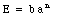The flux through this surface is thus equal to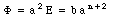This is thus equal to the total flux going through the surface of the cube.

Gauss' law allows us to use the electric flux to calculate the total charge that is enclosed by this Gaussian surface: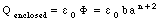Problem 9

For a < r < b, the enclosed charge is equal to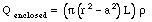The electric field at a distance r is thus equal to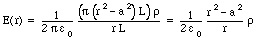Problem 10

For b < r, the enclosed charge is equal to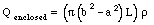The electric field at a distance r is thus equal to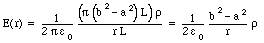Problem 11

This problem can be solved using the principle of superposition. Consider first a solid sphere of radius R and charge Q. The electric field generated by this charge distribution at point P is equal to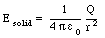Now consider a second sphere with a charge density, identical in magnitude but of opposite sign, and a diameter R, located a distance R/2 from the center of the solid sphere. The electric field produced by this sphere at P is equal to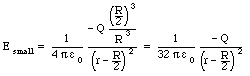The superposition of these two charge distributions produces the charge distribution shown in this problem. The electric field generated by this charge distribution is thus the superposition of the electric fields generated by each of these two charge distributions separately.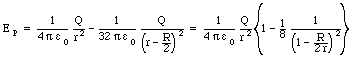Problem 12

The electric field inside the spherical shell is only determined by the point charge at the center of the shell. This most easily can be seen by using a spherical Gaussian surface of radius r (r < a). The enclosed charge is equal to q, and the magnitude of the electric field on the surface of the Gaussian sphere is thus equal to that of a point charge: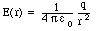Problem 13

The region a < r < b is inside the conductor. The electric field inside any conductor is always equal to 0.

Problem 14

Consider a Gaussian surface of radius r where a < r < b. The electric flux through this surface is equal to 0 (since the electric field on the surface is equal to zero). Thus, the enclosed charge must also be equal to 0. The total enclosed charge is equal to the sum of the point charge at the center of the sphere and the charge located on the inside of the spherical shell. This immediately implies that the total charge on the inside of the spherical shell is equal to -q. The charge density is thus equal to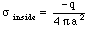Problem 15

Since the spherical shell is uncharged, the charge on the outside surface must be equal to +q. As a consequence, the charge density on the outer surface is equal to